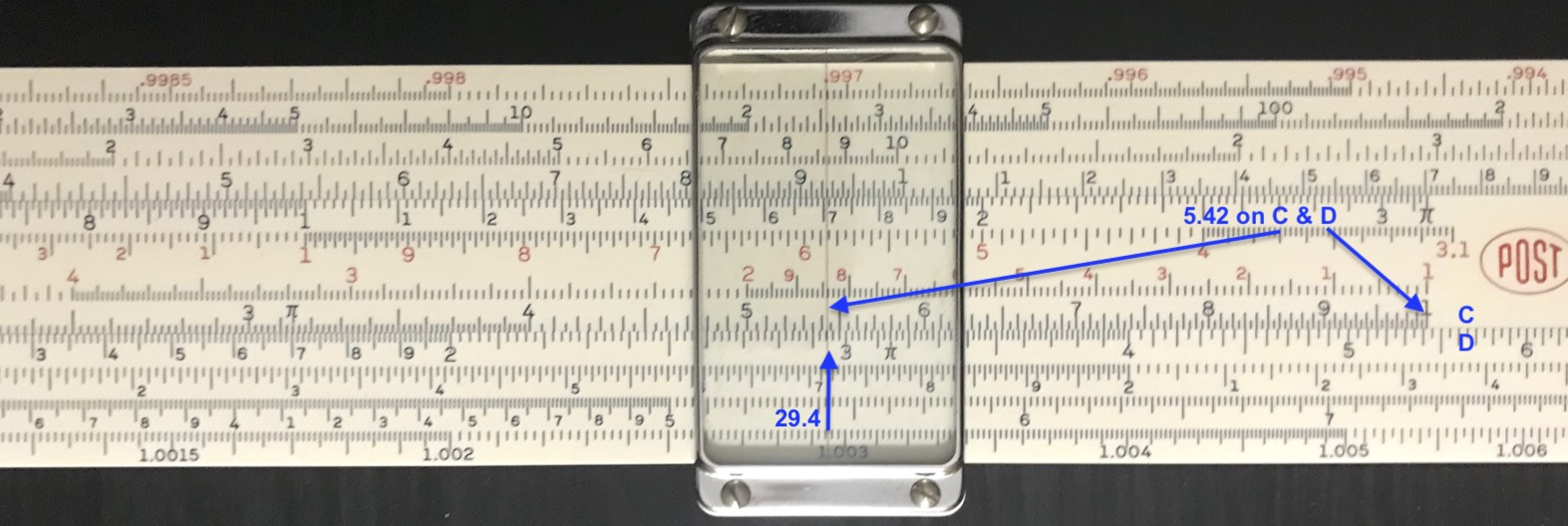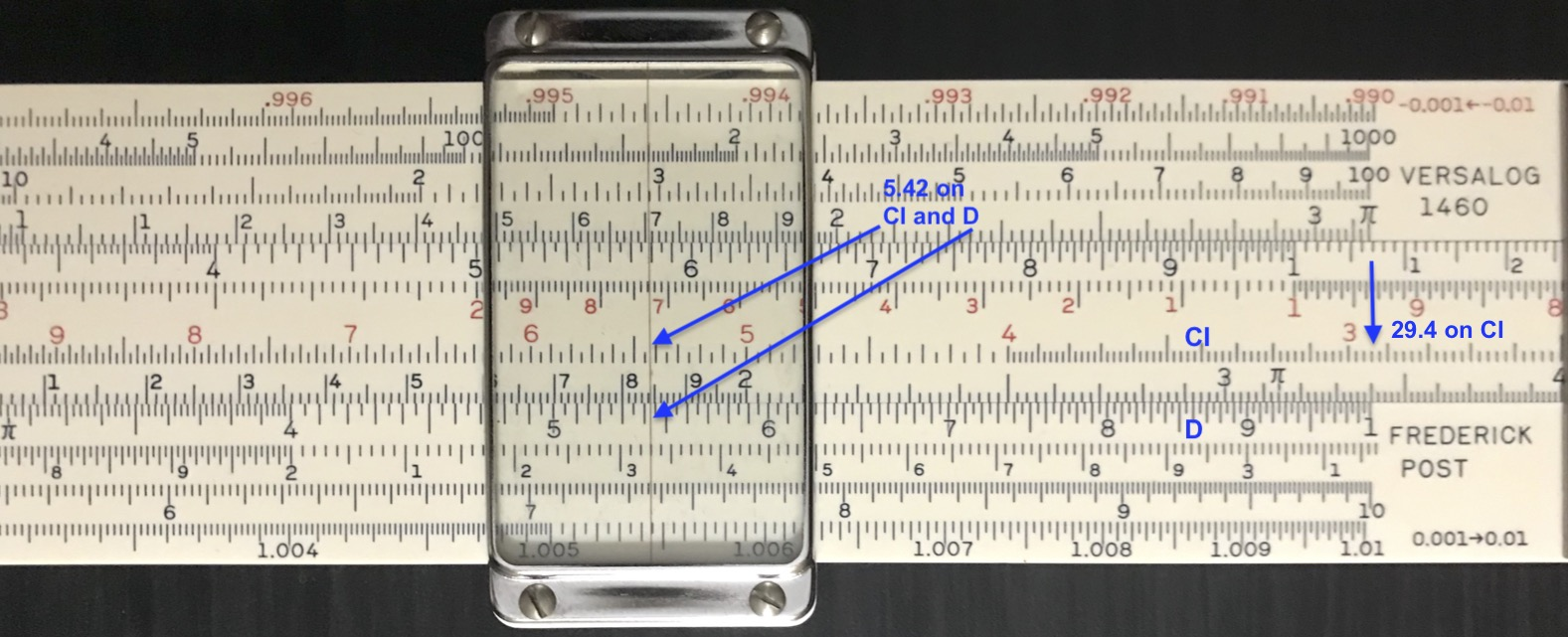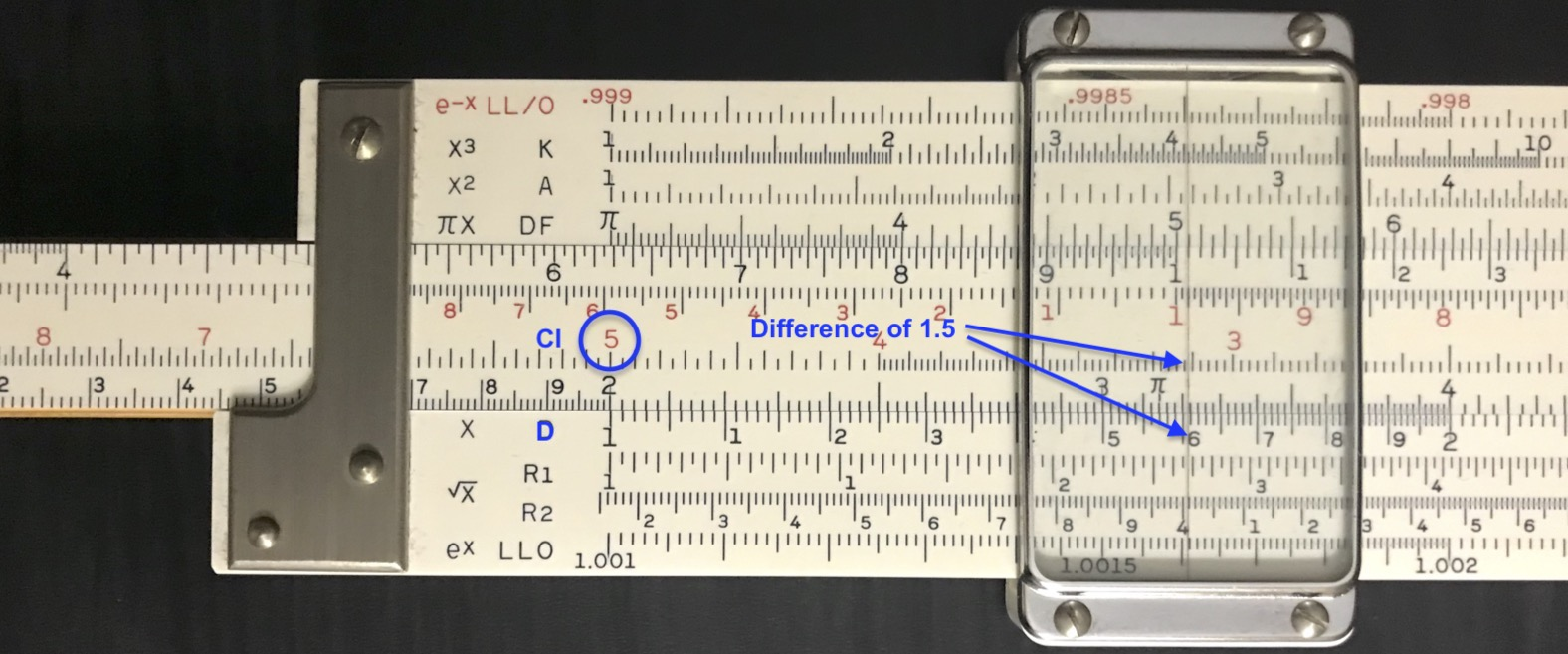## 8.24 Getting to the Root

We are used to using a slide rule to perform simple operations on numbers, such as obtaining values of trigonometric functions, reciprocals and squares and cubes of numbers, and performing relevant multiplication and division calculations and so on. Here we give a standard, but often overlooked technique for solving a general quadratic equation through the use of a slide rule.

Consider the general quadratic equation of variable $$x$$, expressed as

$a x^2 + b x + c = 0$

where the coefficients $$a$$, $$b$$, and $$c$$ are real numbers. It can be shown that for the case where the combination (called the discriminant) $$b^2 - 4ac > 0$$, then two real solutions exist that satisfy the above equation. We will look at a couple of examples of where such solutions can be found using the slide rule.

Square Root with C/D Scales

One often obtains the square root of a number using a Mannheim slide rule, for example, by locating the number on the A scale and reading its square root on the D scale. But what if for some reason there were no A/B scales. We can still solve the problem using the C and D scales alone. So let’s solve for the positive root of $$x^2 = c$$, or $$x=\sqrt{c}$$, using the C and D scales:

• Move the cursor to $$c$$ on the D scale.
• Next, move the slide until the number on C under the cursor is the same as the number on D under the C index. As the rule is now set to multiply a number by the same number, this “same number” is $$x$$!
Here is a calculation of the square root of 29.4:Finding square root of number using C and D scales.

By computer, the square root of 29.4 is 5.422.

$$~$$

Square Root with D and CI Scales

Next, note that $x^2 = c \longrightarrow x = c/x$ and hence $\log x = \log c + \log(1/x), ~~~ or ~~~ \log x - \log(1/x) = \log c.$

So, if there happens to be a CI scale on the slide of the rule, then:

• Align the index of CI with the number $$c$$ on the D scale. Or, equivalently, align the number $$c$$ on the CI scale with the index on the D scale. The two operations should give the same setting of the slide.
• Visually scan the two scales D and CI using the cursor to find where the same number on each lines up. This “same number” is, again, $$x$$. In this case, the rule is set to divide $$x$$ by $$1/x$$ to produce the value $$c$$! In our previous example, note that if 29.4 is on CI at the D index, then 29.4 will also be on the D scale at the CI index.Finding square root of number using D and CI scales.

If there is no CI scale on your slide rule, you can perhaps remove and re-insert the C scale upside down to produce the same effect.

$$~$$

Now take the general quadratic equation we started with at the beginning of this story. It can always be reduced to a form where the coefficient of $$x$$ is equal to 1 (i.e., by dividing through by $$a$$) and hence another form of the quadratic equation would be

$x^2 + gx +h = 0$

where $$g=b/a$$ and $$h=c/a$$. The solutions to this equation will be the same as the solutions to our original equation.

To solve this new equation on a slide rule, we will use the D and CI scales. (Again, the C scale can be reversed if there is no CI scale on the rule.) First, we note that this equation can also be written in terms of its solutions $$u$$ and $$v$$ in the following way, $(x-u)(x-v) = 0$ from which $x^2 -(u+v)x + uv = 0.$ Comparing to our original form, we see that $$u+v = -g$$ and $$u\times v = h$$. So the strategy is to search for two numbers $$u$$ and $$v$$ that satisfy these two conditions:

• Move the slide to align the number $$h$$ on the CI scale with the index on the D scale.
• Move the cursor to where the numbers on the CI and D scales add or subtract to give $$g$$.
• These two numbers are $$u$$ and $$v$$, where a possible minus sign may need to be inferred.

The technique may not be so “automatic”, but with a little practice and patience (as it may take a few iterations to find this special combination) the solution can be found on the rule.

For example, let’s solve the following: $$2x^2 + 3x - 10 = 0$$.

• First, check the discriminant: $$b^2 - 4ac$$ = $$3^2 -4\times 2\times(-10)$$ = $$9+80 >0.~~~~$$ OK. We can do this!.
• Next, we divide everything by 2 to get: $$x^2 + 1.5x - 5 = 0$$.
• Now move the slide to align 5 on the CI scale with the index on the D scale.
• Since $$h$$ in our equation is negative, then one of our two roots will be positive and one will be negative. Thus, we will be looking for two numbers that subtract to make our value of $$-g$$ = 1.5.
• Sliding the cursor, we look for numbers on the CI and D scales at the cursor that add or subtract to 1.5. As expected, we don’t see any numbers adding to 1.5, but a close possibility of a difference of 1.5 is found near the combination of 1.6 and 3.1.
• These two numbers do not quite line up, but a slight tweak of the cursor finds that we can see a more accurate combination that appears to line up: 1.61 and 3.11.
• Since $$g = -(u+v)$$ is $$+1.5$$ in our equation, then the larger of our two numbers must be the negative one, and so our two solutions must be $$x$$ = -3.11 and +1.61.

Here are the roots to our original equation, via computer,

# coeff's for this function are listed in order "c, b, a"
Re(polyroot( c(-10, 3, 2) ))  
##   1.608495 -3.108495Solutions to quadratic equation on slide rule.

Similar techniques using A, K, and BI scales can even be used to solve certain classes of cubic and higher-order equations. Discussions of such techniques can be found through standard on-line searches.